# 数据分析入门系列教程-决策树原理

2020/10/28 08:30

↑ 关注 + 星标 ，每天学Python新技能
后台回复【大礼包】送你Python自学大礼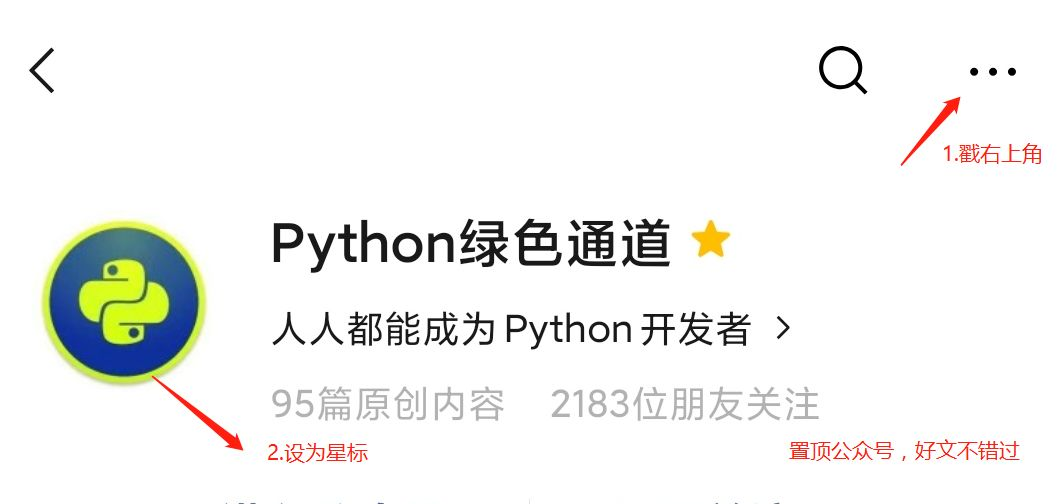# 决策树原理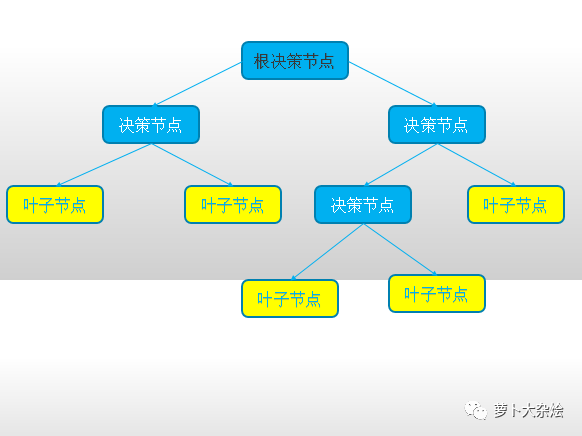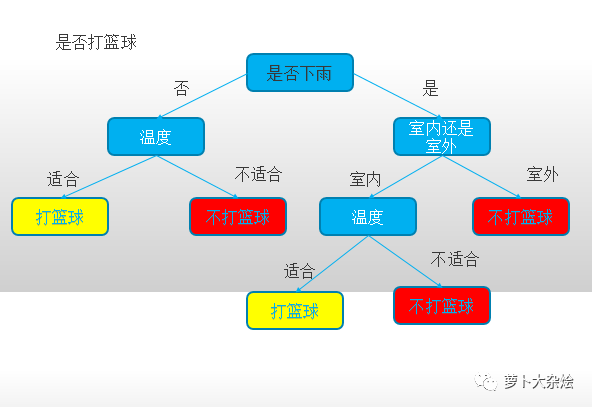## 特征选择

### 信息熵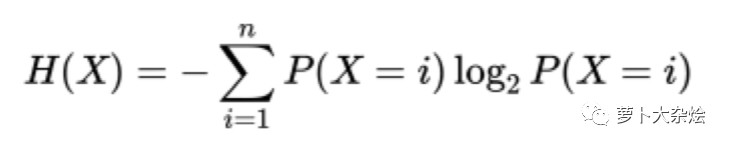P(X=i)为随机变量 X 取值为 i 的概率

n 代表事件的 n 种情况

### 条件熵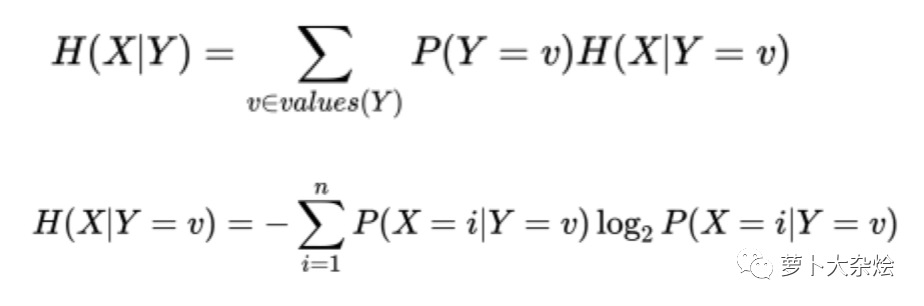Y 为条件

P(Y = v)表示 Y 有多种情况，当 Y 处于 v 的概率

H(X|Y = v)表示 Y 处于 v 条件时的信息熵

### 信息增仪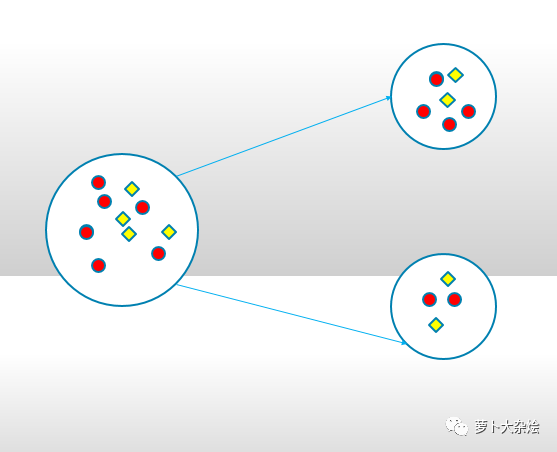= 6/10 * 0.91 + 4/10 * 1

= 0.946

= 0.97 - 0.946

= 0.024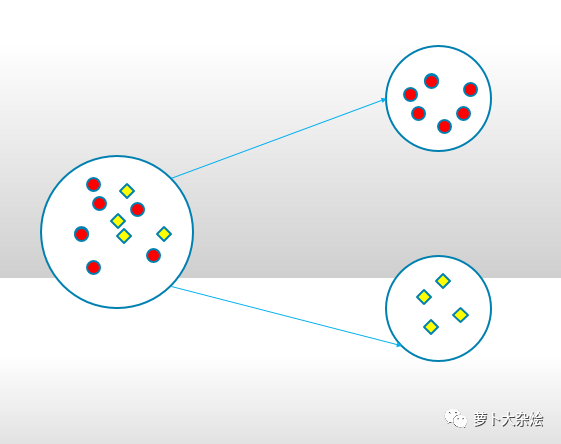## 决策树生成

### 基于 ID3 算法

ID3 算法其实就是计算信息增益，在决策树各个结点上对应信息增益准则（选取信息增益最大的）选择特征，递归地构建决策树。

weather temp wind play golf
rainy hot false no
rainy hot ture no
overcast hot false yes
sunny mild false yes
sunny cool false yes
sunny cool ture no
overcast cool ture yes
rainy mild false no
rainy cool false yes
sunny mild false yes
rainy mild ture yes
overcast mild ture yes
overcast hot false yes
sunny mild ture no

No 5 5/14 = 0.36 -0.531
Yes 9 9/14 = 0.64 -0.410

H(play golf) = -[-0.531 + (-0.410) ] = 0.940

weather Yes No 天气状况各情况出现频度 概率
rainy 2 3 5 5/14 = 0.36
overcast 4 0 4 4/14 = 0.29
sunny 3 2 5 5/14 = 0.36

weather Yes（打高尔夫的概率） No（不打高尔夫的概率） 信息熵
rainy 2/5 = 0.4 3/5 = 0.6 0.971
overcast 4/4 = 1 0/4 = 0 0
sunny 3/5 = 0.6 2/5 = 0.4 0.971

temp Yes No 温度个情况出现频度 概率
hot 2 2 4 4/14 = 0.29
mild 4 2 6 6/14 = 0.43
cool 3 1 4 4/14 = 0.29

temp Yes（打高尔夫的概率） No（不打高尔夫的概率） 信息熵
hot 2/4 = 0.5 2/4 = 0.5 1
mild 4/6 = 0.67 2/6 = 0.33 0.918
cool 3/4 = 0.75 1/4 = 0.25 0.811

wind Yes No 风力各情况出现频度 概率
false 6 2 8 8/14 = 0.57
ture 3 3 6 6/14 = 0.43

wind Yes（打高尔夫的概率） No（不打高尔夫的概率） 信息熵
false 6/8 = 0.75 2/8 = 0.25 0.811
ture 3/6 = 0.5 3/6 = 0.5 1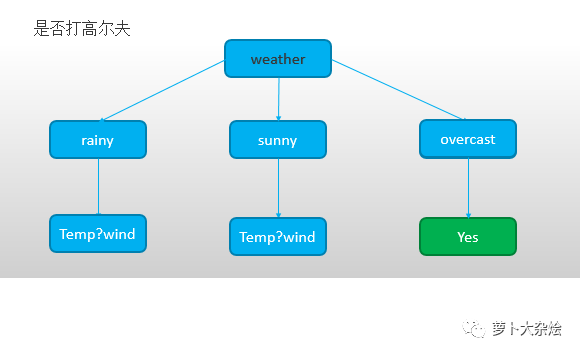sunny 打高尔夫频度 概率 信息熵
Yes 3 3/5 = 0.6 -0.442
No 2 2/5 = 0.4 -0.529

wind Yes No 风力各情况出现频度 概率
false 3 0 3 3/5 = 0.6
ture 0 2 2 2/5 = 0.4

wind Yes（打高尔夫的概率） No（不打高尔夫的概率） 信息熵
false 3/3 = 1 0/3 = 0 0
ture 0/2 = 0 2/2 = 1 0

temp Yes No 风力各情况出现频度 概率
mild 2 1 3 3/5 = 0.6
cool 1 1 2 2/5 = 0.4

temp Yes（打高尔夫的概率） No（不打高尔夫的概率） 信息熵
mild 2/3 = 0.67 1/3 = 0.33 0.915
cool 1/2 = 0.5 1/2 = 0.5 1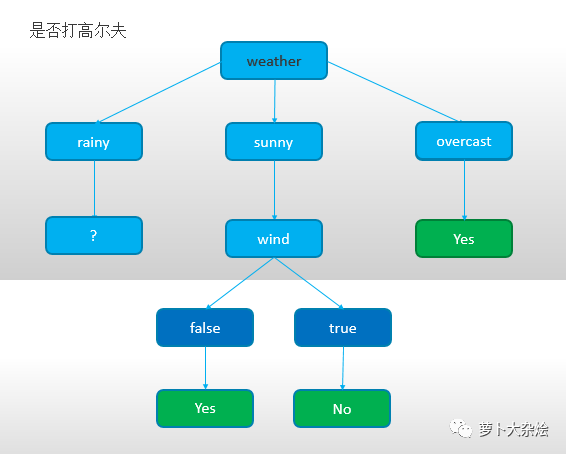rainy 打高尔夫频度 概率 信息熵
Yes 2 2/5 = 0.4 -0.529
No 3 3/5 = 0.6 -0.442

wind Yes No 风力各情况出现频度 概率
false 1 2 3 3/5 = 0.6
ture 1 1 2 2/5 = 0.4

wind Yes（打高尔夫的概率） No（不打高尔夫的概率） 信息熵
false 1/3 = 0.33 2/3 = 0.67 0.915
ture 1/2 = 0.5 1/2 = 0.5 1

temp Yes No 风力各情况出现频度 概率
hot 0 2 2 2/5 = 0.4
mild 1 1 2 2/5 = 0.4
cool 1 0 1 1/5 = 0.2

temp Yes（打高尔夫的概率） No（不打高尔夫的概率） 信息熵
hot 0/2 = 0 2/2 = 1 0
mild 1/2 = 0.5 1/2 = 0.5 1
cool 1/1 = 1 0/1 = 0 0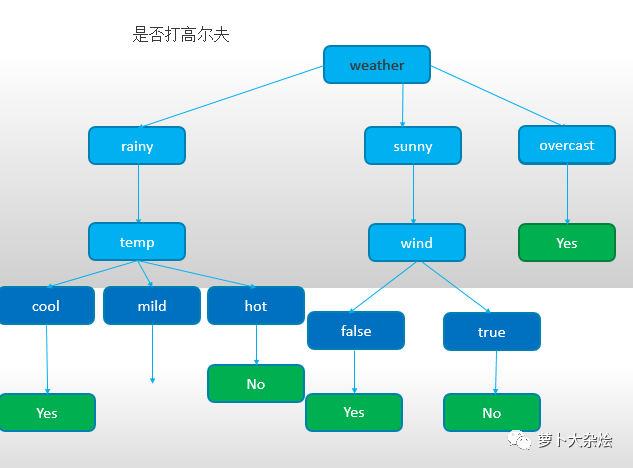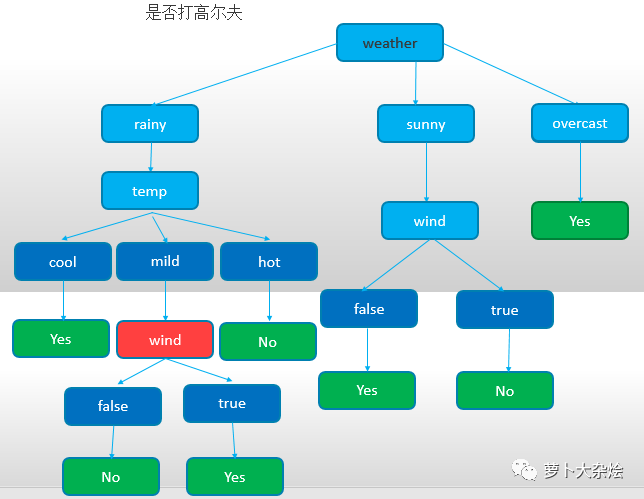### 基于 CART 算法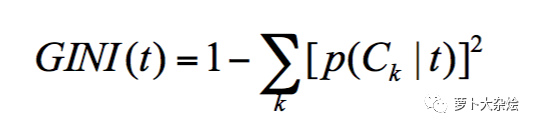p(Ck|t) 表示节点 t 属于类别 Ck 的概率

# 总结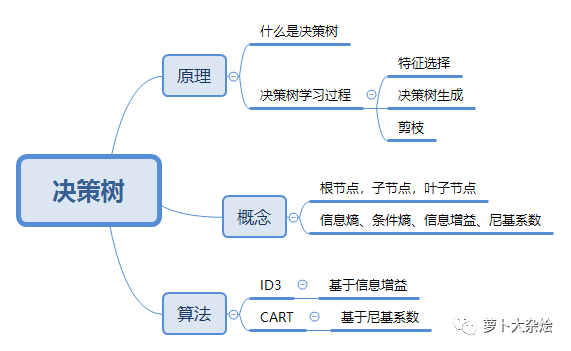# 练习题

1
2
3
4
5
6


-End-勾搭龙哥1. 扫码加我微信备注「资料包」可以领取10本自学电子书，还能围观朋友圈2. 和出版社合作，计划每周送书15本，每月送书50本。3. 白嫖网络课程活动推荐阅读

百度网盘突然大调整，网友炸了！！

前百度算法工程师带，你深度掌握NLP学习进阶方法~

那个火遍全球后被封杀的“AI一键脱衣”软件，又卷土重来了

修改微信（支付宝）运动步数，轻松 TOP1扫码回复「大礼包」后获取大礼



0
0 收藏

### 作者的其它热门文章0 评论
0 收藏
0